IntroductionIntroductionINTRO
When we’re asked to find the area of a quadrilateral, what we’re really looking for is the amount of space inside the boundaries or sides that connect to make the shape.
Let’s imagine we want to build a small field in a Minecraft world and need to figure out the amount of land (area) in a 3 × 5 field.
We can start with a strip of land that has blocks of grass.
CALCULATOR

#### Step 1. Identify the length & width of the rectangle.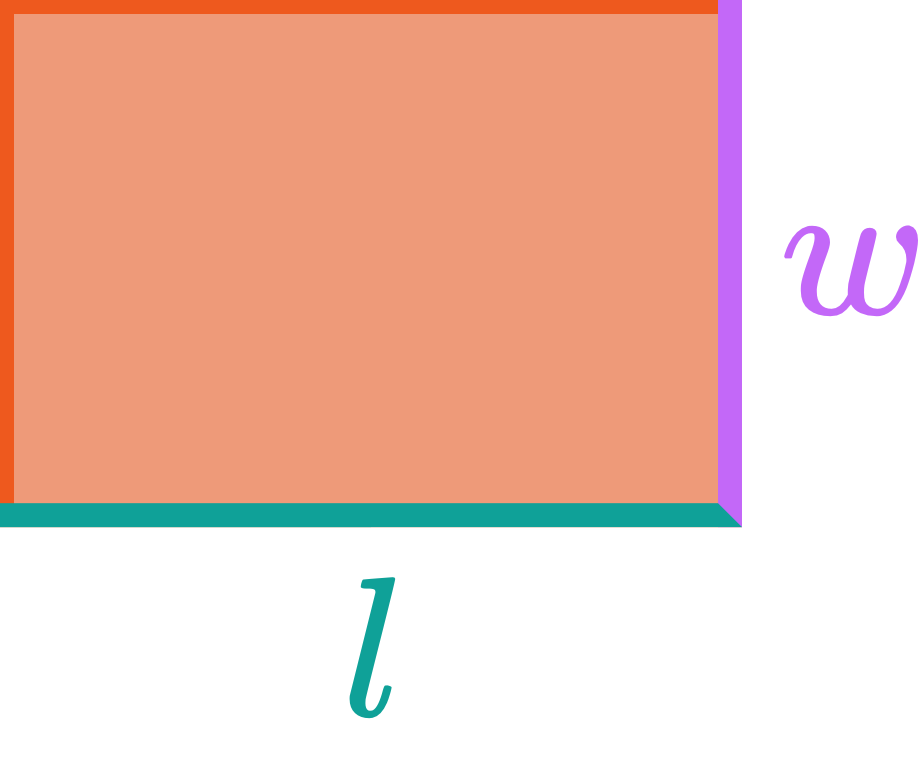KEY STEPS

## How to Find the Area of a Quadrilateral

### Step 1. Identify the length & width of the rectangle.### Step 2. Multiply the length by the width.

LESSON
Area of Rectangles & Squares

## Area of Rectangles & Squares

The formula for the area of a rectangle is , where is the length of the rectangle and is the width.

We can use the same formula for finding the area of a square.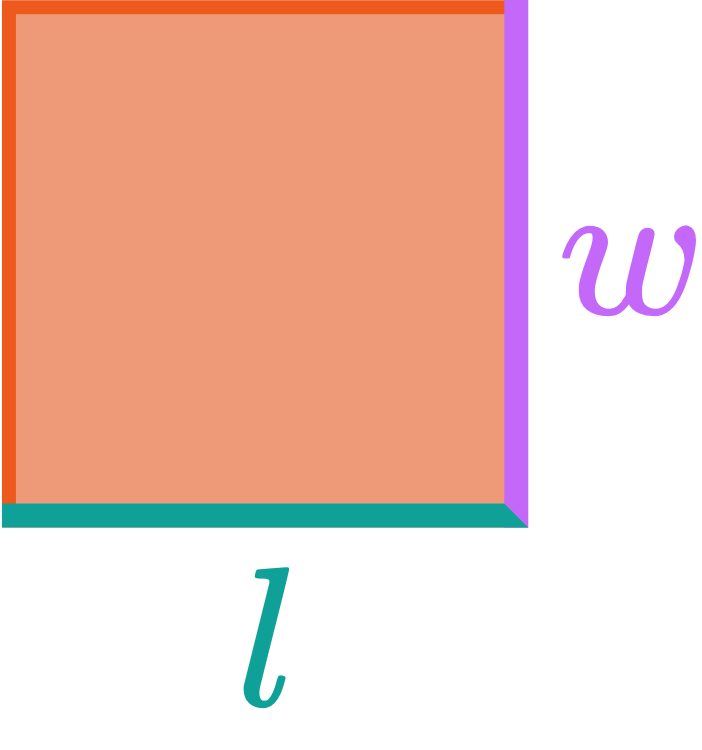Since all the side lengths are equal in a square, , we can also get the area of a square using , where is the side of the square.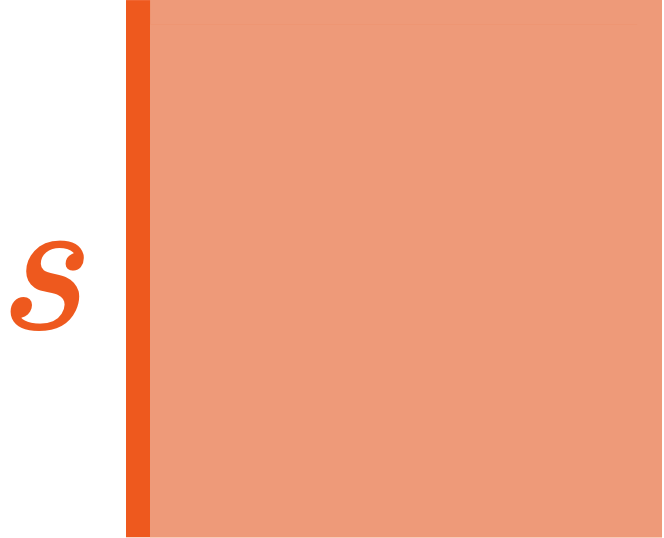PRACTICE
Area of Rectangles & Squares

## Practice: Area of Rectangles & Squares

Question 1 of 4: Find the area of this quadrilateral ⬇️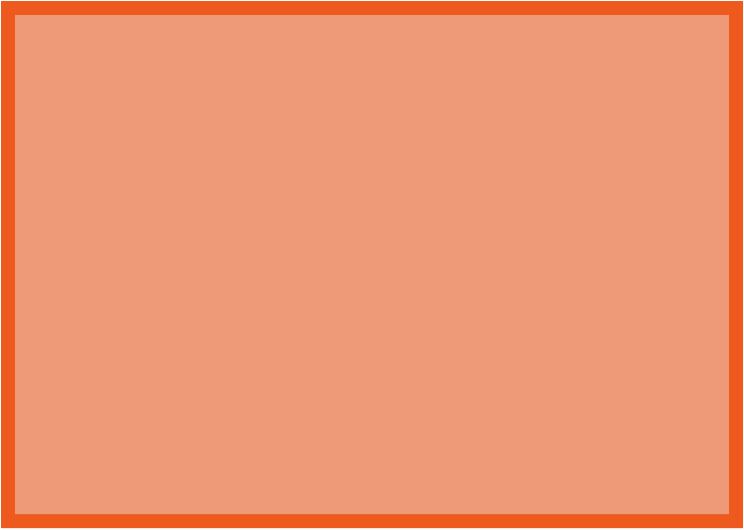#### Step 1. Identify the length & width of the rectangle.

LESSON
Area of Parallelograms

## Area of Parallelograms

The formula for the area of a parallelogram is . Notice how it looks a lot like the formula for the area of a rectangle .

That’s because a parallelogram can be rearranged to form a rectangle: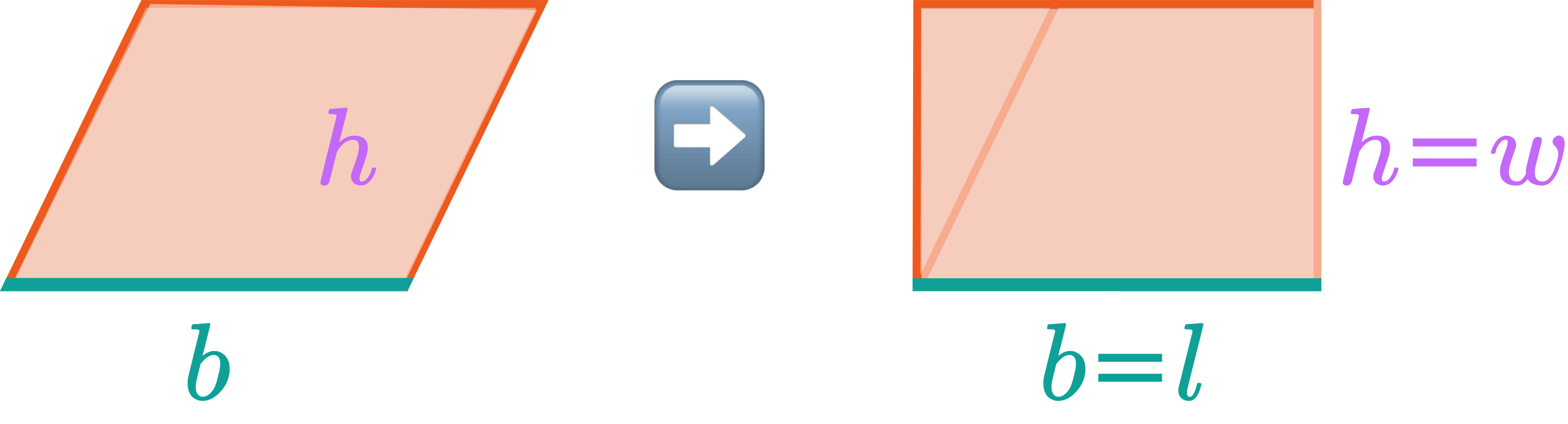The base of a parallelogram corresponds to the length of a rectangle, and the height of a parallelogram corresponds to the width of a rectangle.
Let’s take a look at an example of how to solve for the area of a parallelogram.
PRACTICE
Area of Parallelograms

## Practice: Area of Parallelograms

Question 1 of 4: Find the area of this quadrilateral ⬇️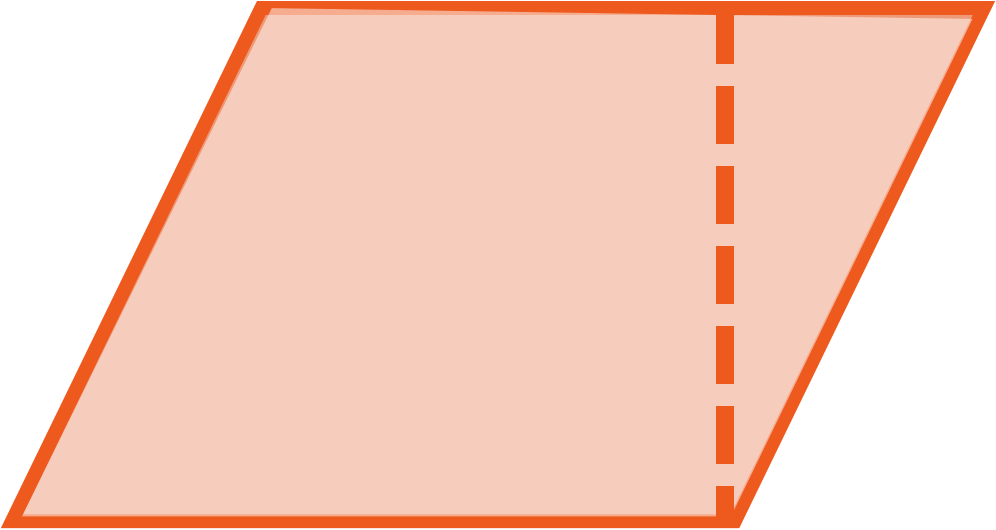#### Step 1. Identify the base & height of the parallelogram.

LESSON
Area of Rhombuses & Kites

## Area of Rhombuses & Kites

The formula for the area of a parallelogram is .

It may not be obvious at first, but if we split a kite or rhombus into two identical pieces, we can rearrange them to form a parallelogram with a base equal to , and a height equal to :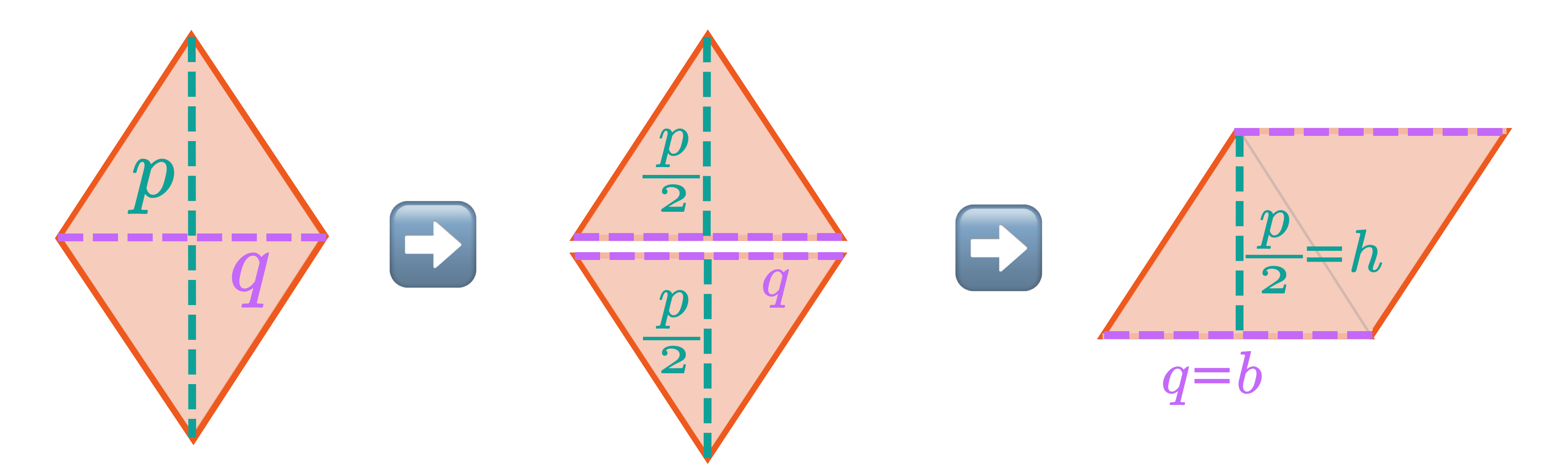Let’s take a look at an example of how to solve for the area of a rhombus.
PRACTICE
Area of Rhombuses & Kites

## Practice: Area of Rhombuses & Kites

Question 1 of 4: Find the area of this quadrilateral ⬇️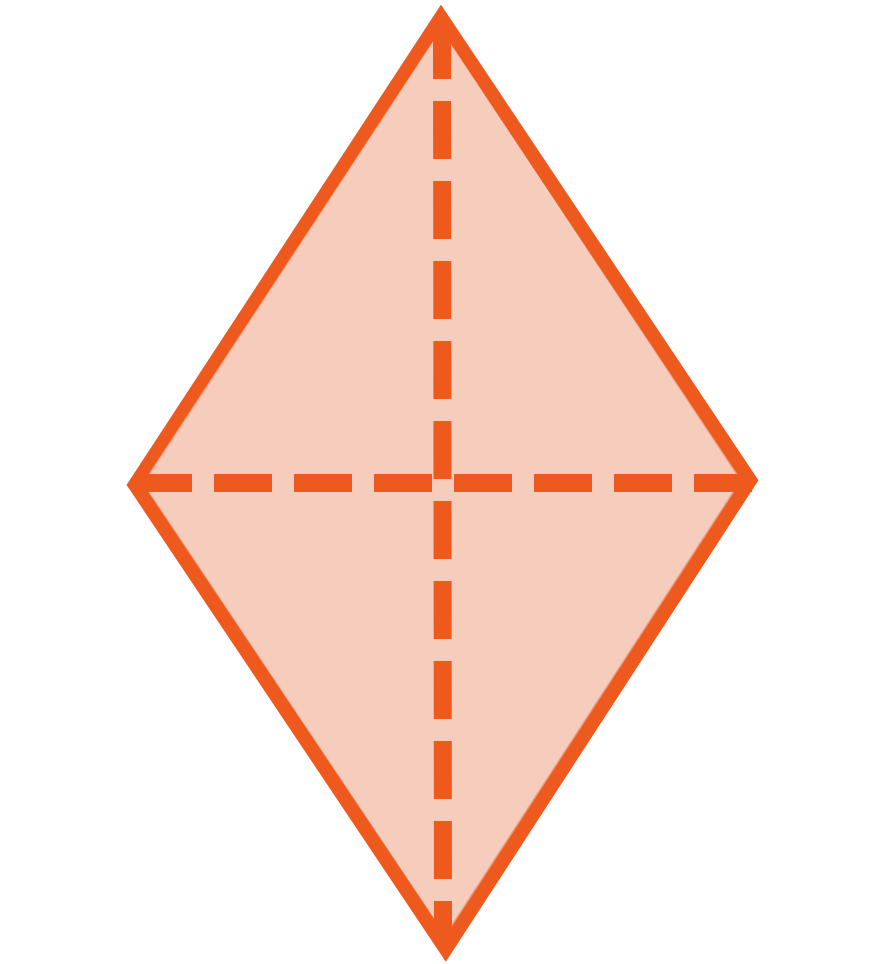#### Step 1. Identify the diagonal/crosslengths of the rhombus.

LESSON
Area of Trapezoids

## Area of Trapezoids

The formula for the area of a trapezoid is .

It may not be obvious at first, but if we have two identical trapezoids, we can rearrange them to form a parallelogram with a base equal to , and a height equal to :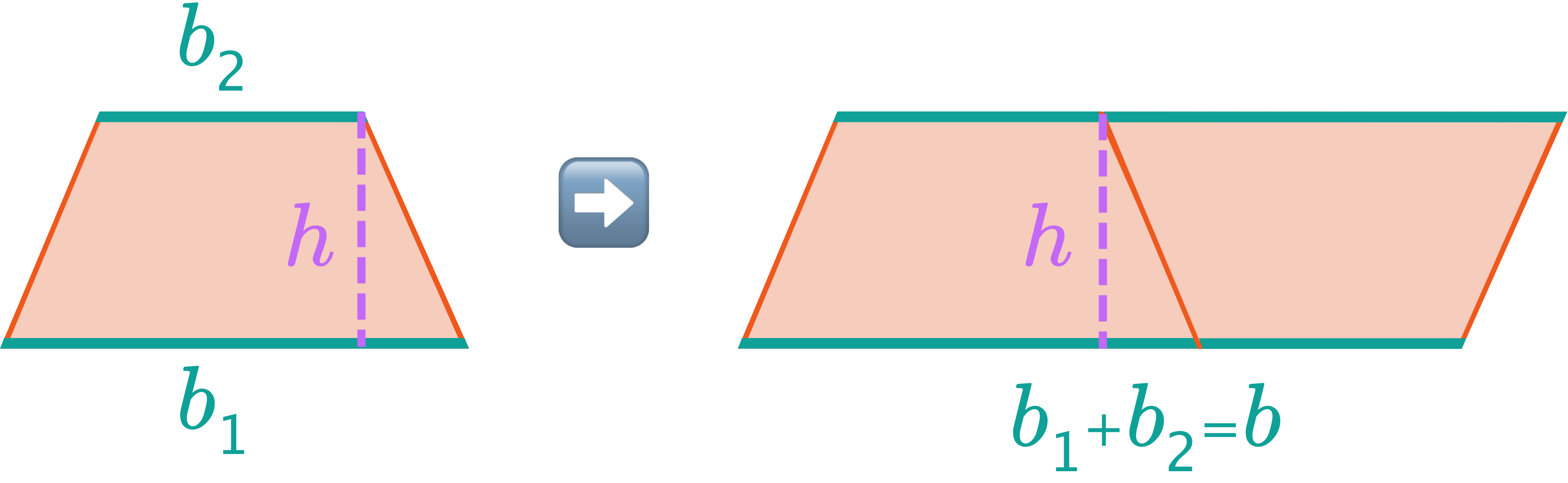Let’s take a look at an example of how to solve for the area of a trapezoid.
PRACTICE
Area of Trapezoids

## Practice: Area of Trapezoids

Question 1 of 4: Find the area of this quadrilateral ⬇️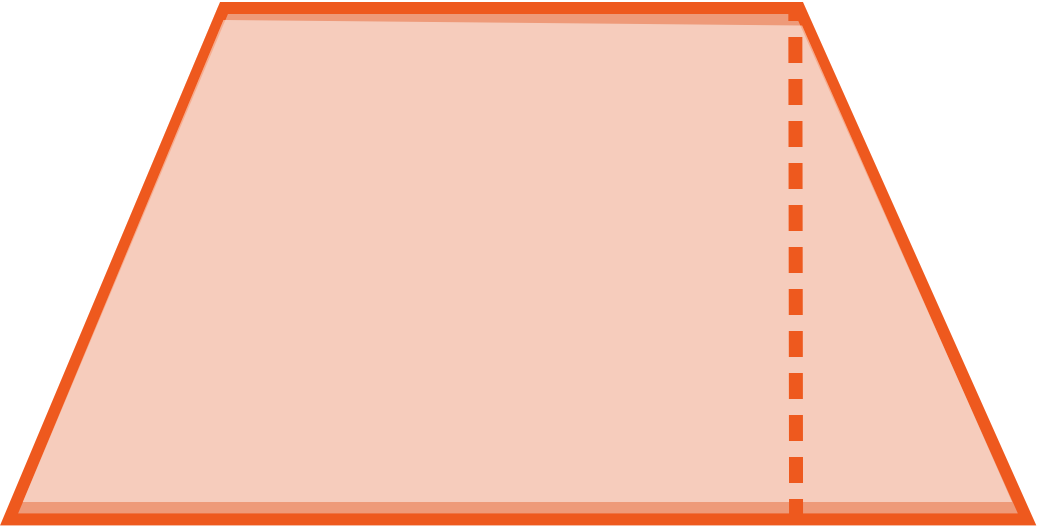#### Step 1. Identify the base lengths and height of the trapezoid.

CONCLUSION
Incredible work, look at you go! Thanks for checking out this lesson ☺️🙏. Where to next?Leave Feedback